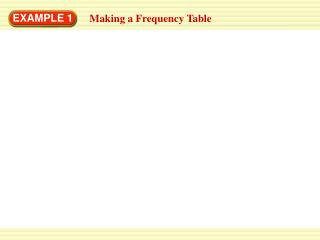DownloadDownload PresentationEXAMPLE 1

# EXAMPLE 1

Download Presentation## EXAMPLE 1

- - - - - - - - - - - - - - - - - - - - - - - - - - - E N D - - - - - - - - - - - - - - - - - - - - - - - - - - -
##### Presentation Transcript

1. EXAMPLE 1 Making a Frequency Table

2. EXAMPLE 1 Making a Frequency Table To find which type of art project was chosen most often, you can make a frequency table.

3. EXAMPLE 1 Making a Frequency Table To find which type of art project was chosen most often, you can make a frequency table.

4. EXAMPLE 1 Making a Frequency Table To find which type of art project was chosen most often, you can make a frequency table.

5. EXAMPLE 1 Making a Frequency Table To find which type of art project was chosen most often, you can make a frequency table.

6. EXAMPLE 1 Making a Frequency Table To find which type of art project was chosen most often, you can make a frequency table. ANSWER The students most often chose a painting project.

7. for Example 1 GUIDED PRACTICE • The data at the top of the page could have been recorded in a frequency table as the information was gathered. Explain how this could be done and why this might be a better way to record the data.

8. for Example 1 GUIDED PRACTICE • What If? Suppose the choices for 5 more students were: sculpture, sculpture, drawing, drawing, sculpture. Make a new frequency table including these data. Which type of project was chosen most often?

9. EXAMPLE 2 Making a Line Plot

10. EXAMPLE 2 Making a Line Plot Summer Reading The frequency table shows how many books the students in a class read during summer vacation.

11. EXAMPLE 2 Making a Line Plot Summer Reading The frequency table shows how many books the students in a class read during summer vacation. • Make a line plot of the data.

12. EXAMPLE 2 Making a Line Plot Summer Reading The frequency table shows how many books the students in a class read during summer vacation. • Make a line plot of the data. • Use the line plot to find the total number of students.

13. EXAMPLE 2 Making a Line Plot Summer Reading The frequency table shows how many books the students in a class read during summer vacation. • Make a line plot of the data. • Use the line plot to find the total number of students. • Use the line plot to find how many students read four or more books.

14. EXAMPLE 2 Making a Line Plot SOLUTION

15. EXAMPLE 2 Making a Line Plot SOLUTION • Make a line plot of the data.

16. EXAMPLE 2 Making a Line Plot SOLUTION • Make a line plot of the data.

17. EXAMPLE 2 Making a Line Plot SOLUTION • Make a line plot of the data.

18. EXAMPLE 2 Making a Line Plot SOLUTION • Make a line plot of the data. • There are 22 x marks in all, so the total number of students is 22.

19. EXAMPLE 2 Making a Line Plot SOLUTION • Make a line plot of the data. • There are 22 x marks in all, so the total number of students is 22. • The total number of x marks above the numbers 4, 5 and 6 is six, so six students read four or more books.

20. GUIDED PRACTICE for Example 2 MULTIPLE REPRESENTATIONS The following data show the numbers of letters in students’ names. Use the data in Exercises 3–5. 6, 5, 4, 4, 5, 3, 9, 8, 6, 4, 3, 4, 7, 5, 4, 3, 8, 4, 9, 3 • Make a frequency table of the data. • Make a line plot of the data. • Choose one of the displays. Use it to find out whether more students have names with 3 letters or names with 7 or more letters. Describe which display you choose and how you use it to answer the question.